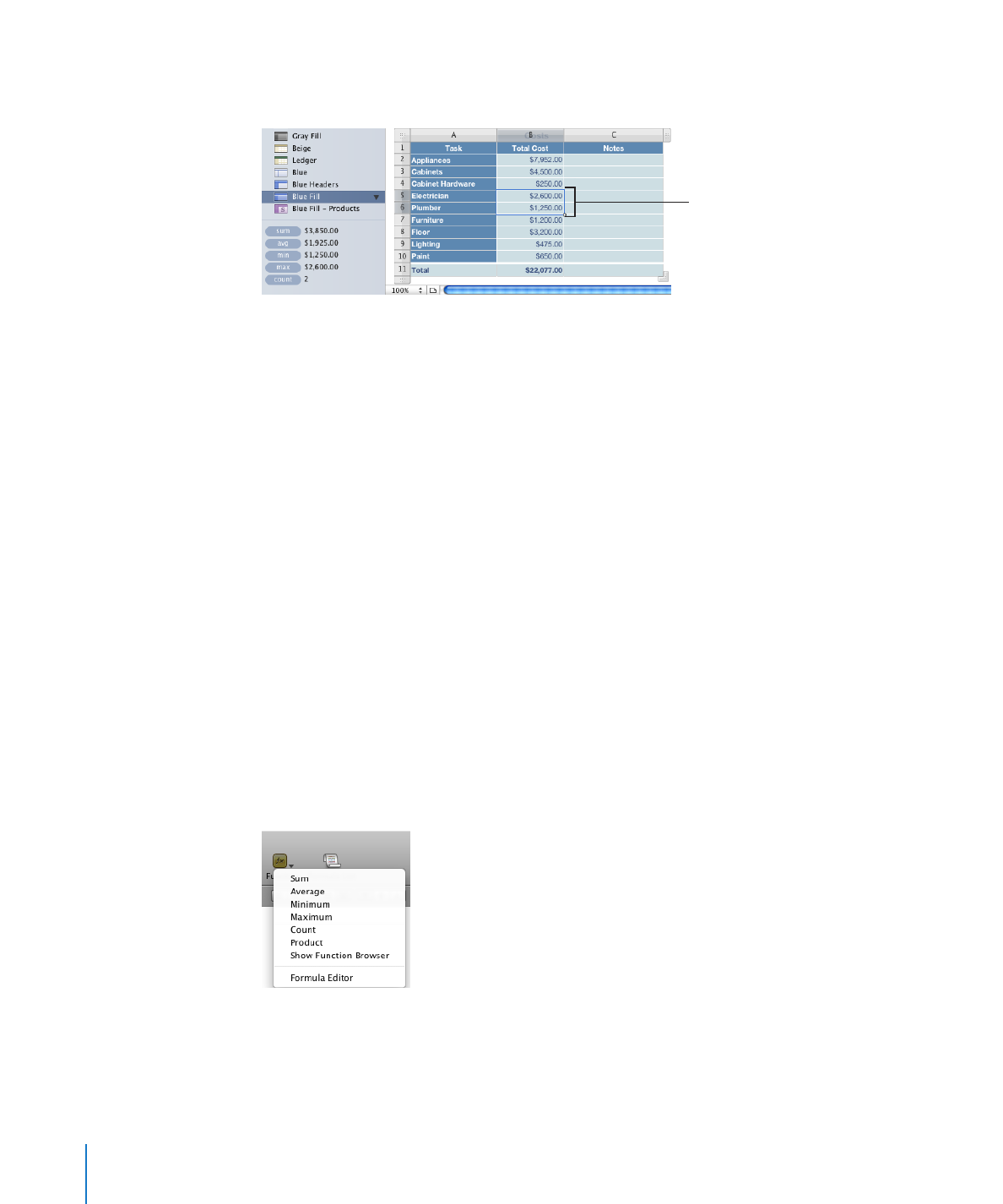## Numbers - Using Predefined Quick FormulasUsing Predefined Quick Formulas

An easy way to perform a basic calculation using values in a range of adjacent table
cells is to select the cells and then add a quick formula using the pop-up menu visible
when you click Function in the toolbar.

Sum: Calculates the sum of numeric values in selected cells.
Average: Calculates the average of numeric values in selected cells.
Minimum: Determines the smallest numeric value in selected cells.

120

Chapter 6

Using Formulas in TablesChapter 6

Using Formulas in Tables

121

Maximum: Determines the largest numeric value in selected cells
Count: Determines the number of numeric values and date/time values in
selected cells.
Product: Multiplies all the numeric values in selected cells.

You can also choose Insert > Function and use the submenu that appears.

Empty cells and cells containing types of values not listed are ignored.

Here are ways to add a quick formula:
To use selected values in a column or a row, select the cells, click Function in the

m

toolbar, and then choose a calculation from the pop-up menu.
If the cells are in the same column, Numbers places the formula in the first empty
cell beneath the selected cells. If there is no empty cell, Numbers adds a row to hold
the formula.
If the cells are in the same row, Numbers places the formula in the first empty cell to
the right of the selected cells. If there is no empty cell, Numbers adds a column to hold
the formula.
To use

m

all the values in a column’s body cells, click the column’s header cell or

reference tab, click Function in the toolbar, and then choose a calculation from the